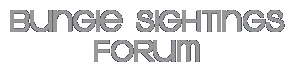## G1 = 41

Posted By: Luigi <lorsina@mac.com>
Date: 17 June 2001, 03:15

I do not know whether or not this has sense, but...

41 = 41 = 1*41
20+62 = 82 = 2*41
24+37+62 = 123 = 3*41
19+20+50+75 = 164 = 4*41
19+20+41+50+75 = 205 = 5*41
19+24+37+41+50+75 = 246 = 6*41
19+20+24+37+50+62+75 = 287 = 7*41
19+20+24+37+41+50+62+75 = 328 = 8*41

Is this the pattern we are looking for?

BTW: how many numbers should any glyph yield? One, or two?

Luigi